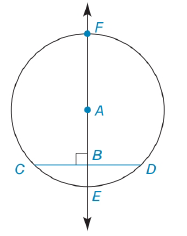Chapter 6.3, Problem 44E### Elementary Geometry for College St...

6th Edition
Daniel C. Alexander + 1 other
ISBN: 9781285195698

#### Solutions

Chapter
Section### Elementary Geometry for College St...

6th Edition
Daniel C. Alexander + 1 other
ISBN: 9781285195698
Textbook Problem
1 views

# In Exercises 44 to 47, prove the stated theorem.If a line is drawn through the center of a circle perpendicular to a chord, then it bisects the chord and its minor arc. See Figure 6.37 on page 288.(NOTE: The major arc is also bisected by the line.)To determine

To find:

To prove the following stated theorem,

“If a line is drawn through the center of a circle perpendicular to a chord, then it bisects the chord and its minor arc”.

Explanation

Given that ABCD¯ in circle A.

To prove CB¯BD¯ and CE^ED^

The diagrammatic representation is given below,

Since ABCD¯ therefore ΔABC=ΔABD=90 that is mABC=mABD=90.

We know that angles which are opposite to equal sides are also equal.

Also, mABC=mABD=90

By using AAS congruence rule ( that is any two angle of one triangle is congruent to corresponding two angles of the other triangles and one side of the one triangle is congruent to the corresponding side of the other triangles then these triangles are congruent)

Using the CPCTC (corresponding parts of congruent triangles are congruent) to get the following,

CB¯BD¯

Consider the ΔACD to get the following,

### Still sussing out bartleby?

Check out a sample textbook solution.

See a sample solution

#### The Solution to Your Study Problems

Bartleby provides explanations to thousands of textbook problems written by our experts, many with advanced degrees!

Get Started